# How to Copy and Paste Values Skipping Duplicates in Excel?

Sometimes in Excel, you want to only copy the values that are unique. The values that are repeated are known as duplicate values. If we try to manually select only the unique values to copy, then it can be a time-consuming and inaccurate process. Read this tutorial to learn how to copy and paste values in Excel while avoiding duplicates.

## Copy and Paste Values Skipping Duplicates Using Filter

Here, we will first select the data and then use the filter to paste the values, skipping the duplicate values. Let us see a simple process to know how we can copy and paste values while skipping duplicates using a filter in Excel.

### Step 1

Consider an excel sheet containing a list of names, similar to the image below.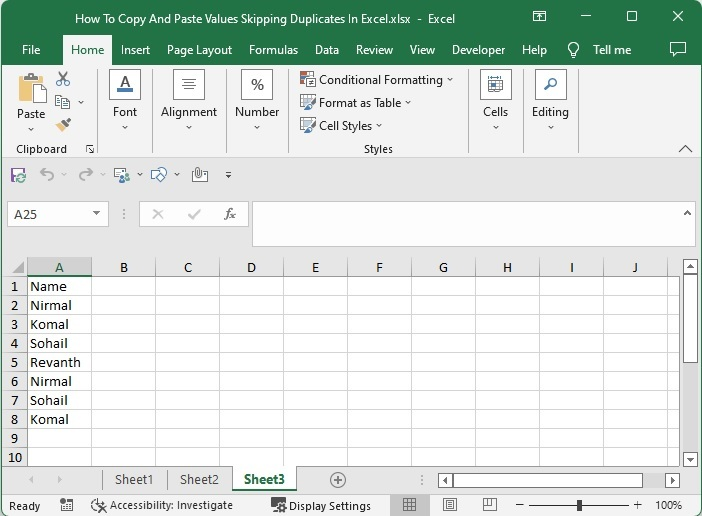Now select the range of data on the sheet, click on data, and select advanced filter to open the pop-up.

Select data > Data > Advanced filter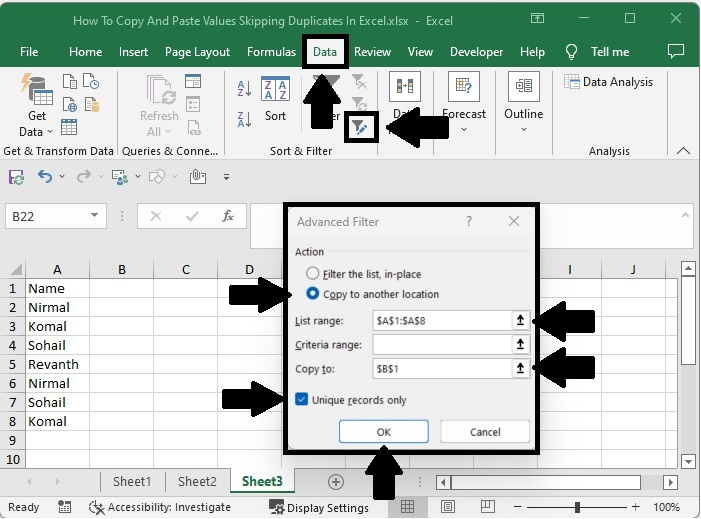### Step 2

In the pop-up window, click on "Copy to Other Location" and specify the location where you want to start the new values, then select the checkbox "Unique Records Only" and click "OK."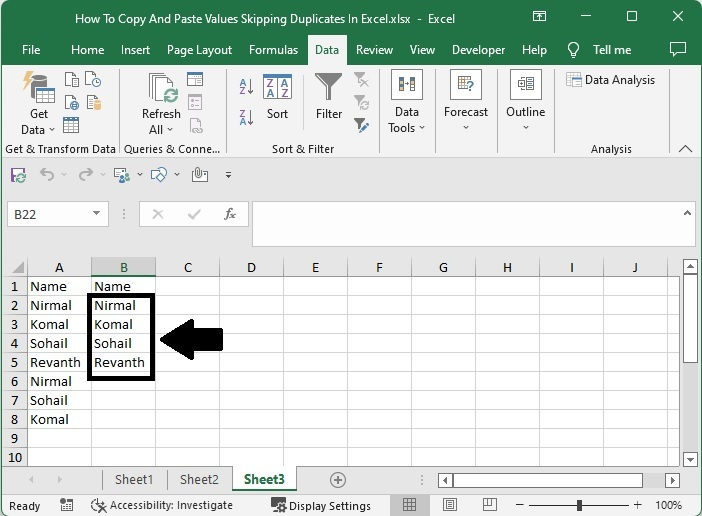## Copy and Paste Values Skipping Duplicates Using Formulas

Here, we will first use the index and match formulas to get the first result, then use the auto-fill handler to fill in all the values. Let us see a simple process to know how we can copy and paste values, skipping duplicates, using formulas in Excel.

### Step 1

Let us consider the same data that we used in the above example.

Then, in our case, click on an empty cell, B2, and enter the formula =INDEX($A$2:$A$8, MATCH(0, COUNTIF($B$1:B1, $A$2:$A$8), 0)) and press CTRL + SHIFT + ENTER to get the first result, which looks like the image below. In the formula, A2:A8 is the range of names on the sheet, and B2 is our current address.

Empty cell > Formula > CTRL + SHIFT + ENTER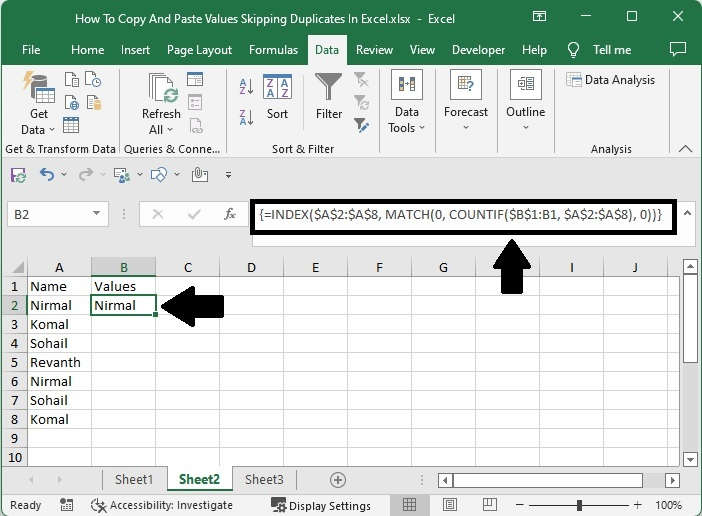### Step 2

To get all the results, drag down from the first result using the auto-fill handle, and our final result will be similar to the below image.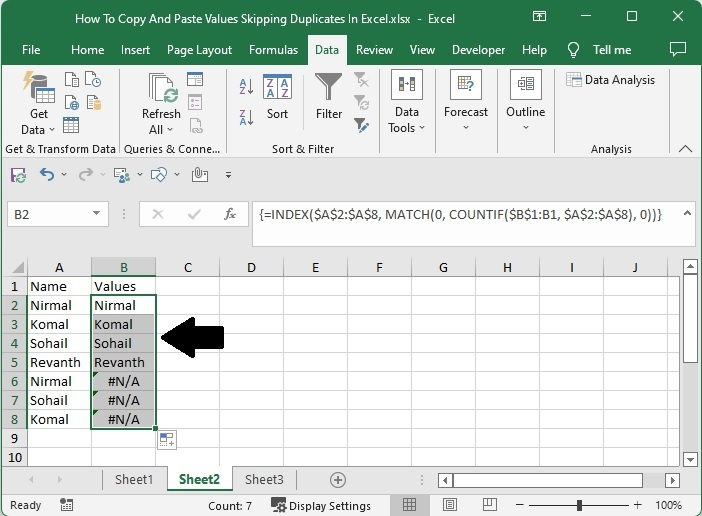## Conclusion

In this tutorial, we used a simple example to demonstrate how we can copy and paste values in Excel, skipping duplicates.